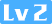python实现——最优化算法(二分法、格点法、黄金分割法、牛顿法等)Souleigh ✨179 0 0

## 二分法

def asdf(x):
rres=8*x**3-2*x**2-7*x+3
return rres

i=2
left=0
right=1
while i>0 :
i = i-1
ans = 0.1
mid1 = (left + right + ans) / 2
mid2 = (left + right - ans) / 2
a=asdf(mid1)
c=asdf(mid2)
if a > c :
right = mid1
else :
left = mid2
b=(left+right) / 2
print("左极限=%s,右极限=%s,极小值x=%s"%(left,right,b)) 
左极限=0.45,右极限=0.775,极小值x=0.6125 

1.def是python中指定义，一般用来定义函数，如果需要深度学习搭建网络可用来定义网络。值得注意的一点是

return必须要加在函数后面另起一行。

2.最坑的就是逻辑。一开始逻辑没理清楚，或者说在代码上有疏漏，导致我将left和right放在了循环体里，结果可想而知。不过也是因为这个错误，我知道pycharm中的debug怎么用，挺简单的，百度一下就出来了。

3.不知道什么原因，看的莫烦视频中的print多个变量一起输出是没有办法在我的pycharm中使用的，出来的结果很奇怪。可能是因为我是win10不是ios吧。print如果多个变量一起输出必须是print("名字：%s,名字2：%s"%(a,b))结果输出就是名字：a ,名字2：b

##### 问题：1.为什么要加return？

return的意思是输出这个def里面任意一个变量值作为结果显示。一般情况而言，是输出函数的关系式的命名，这样当你调用这个函数的时候，变量对应的函数值才能显示出来，否则只运行没有结果，不会有效果。

## 格点法——三点等分法

import numpy as np
def qwer(x):
third = np.exp(x) - 5*x
return third

left = 1
right = 2
mid1 =float(left+right) / 2
mid2 = (left+mid1) / 2
mid3 = (mid1+right) /2
a = qwer(mid1)
b = qwer(mid2)
c = qwer(mid3)
i = 5
while i > 0:
i=i-1
if a > b:
if c > b :
#b
right = mid1
mid1 = mid2
a=b
mid2 = (left + mid1) / 2
mid3 = (mid1 + right) / 2
b = qwer(mid2)
c = qwer(mid3)
else:#b>c
#c
left = mid1
mid1 = mid3
a = c
mid2 = (left + mid1) / 2
mid3 = (mid1 + right) / 2
b = qwer(mid2)
c = qwer(mid3)
else:#b>a
if a > c:
#C
left = mid1
mid1 = mid3
a = c
mid2 = (left + mid1) / 2
mid3 = (mid1 + right) / 2
b = qwer(mid2)
c = qwer(mid3)
else:#b>a&c>a
# a
left = mid2
right = mid3
mid2 = (left + mid1) / 2
mid3 = (mid1 + right) / 2
b = qwer(mid2)
c = qwer(mid3)

print("最小值=%s"%mid1)
print("函数值=%s"%a) 
最小值=1.609375

left = 1.0
right = 2.0
mid1 =(left+right) / 2 

## Fibonacci法

def fibonacci(n):
i=0
a = 0
b = 1
for i in range(n):
i=i+1
c = a+b
a = b
b = c
return c
def bn(x):
ert = x**2 - 6*x + 2
return ert
z = 2
p = 0
left = 0.00000
right = 10.00000
L1 = right - left
while z < 100:
m = fibonacci(z)
l = L1/m
k = 1.000/m
if k < 0.03:
print("n=%s,Fn=%s"%(z,m))
L2 = l*fibonacci(z-1)
t = left + L2
r = right -L2
while p < 3:
p = p + 1
l3 = t - r
e= bn(t)
o = bn(r)
if e>o :
right = t
t = r
r = left + l3
else:#o>e
left = r
r = t
t = right - l3
break
else:
z = z + 1

okk=(left+right)/2
okky=bn(okk)
print(left)
print(right)
print("极小值x=",okk)
print("极小值y=",okky) 

fibonacci函数定义，每次debug后我的手都是抖的O(∩_∩)O~

## 黄金分割法

def gold(x):
gg= x**2 - 6*x + 9
return gg

left = 1
right = 7
ans = 0.4
a = left + 0.618 * (right - left)
b = left + 0.382*(right - left)
gga = gold(a)
ggb = gold(b)
i = 0
while i < 7:
print("i=%s" % i)
print("left=%s,right=%s" % (left, right))
print("x左=%s,x右=%s" % (a, b))
print("y左=%s,y右=%s" % (ggb, gga))
c = right - left
if c > 0.4:
i = i + 1
if gga > ggb:
right = a
a = b
b = left + 0.382*(right - left)
gga = ggb
ggb = gold(b)
else:#gga<ggb
left = b
b = a
a = left + 0.618 * (right - left)
ggb = gga
gga = gold(a)
else:
break 

### 间接法——二次插值法

def yy(x):
y=x**4-4*x**3-6*x**2-16*x+4
return y

def xing(xm1,xm2,xm3,fm1,fm2,fm3):
yxxx=0.5000*((xm2**2-xm3**2)*fm1+(xm3**2-xm1**2)*fm2+(xm1**2-xm2**2)*fm3)/((xm2-xm3)*fm1+(xm3-xm1)*fm2+(xm1-xm2)*fm3)
return yxxx

x1 = -1.0000
f1 = yy(x1)
x3 = 6
f3 = yy(x3)
x2 = 0.50000*(x1+x3)
f2 = yy(x2)
xp = xing(x1,x2,x3,f1,f2,f3)
fp = yy(xp)
a = abs(xp-x2)
while abs(xp-x2) > 0.05000:
a = abs(xp - x2)
if xp > x2:
if fp > f2:
x3=xp
f3=fp
xp = xing(x1, x2, x3, f1, f2, f3)
fp = yy(xp)
print("ans=%s" % a)
print("left=%s,right=%s" % (x1, x3))
print("x*=%s,fp*=%s" % (xp, fp))
print("x2=%s,f2=%s" % (x2, f2))
print("******************")
else:#f2>fp
x1 = x2
f1 = f2
x2 = xp
f2 = fp
xp = xing(x1, x2, x3, f1, f2, f3)
fp = yy(xp)
print("ans=%s" % a)
print("left=%s,right=%s" % (x1, x3))
print("x*=%s,fp*=%s" % (xp, fp))
print("x2=%s,f2=%s" % (x2, f2))
print("******************")
else:#xp<x2
if fp > f2:
x1 = xp
f1 = fp
xp = xing(x1, x2, x3, f1, f2, f3)
fp = yy(xp)
print("ans=%s" % a)
print("left=%s,right=%s" % (x1, x3))
print("x*=%s,fp*=%s" % (xp, fp))
print("x2=%s,f2=%s" % (x2, f2))
print("******************")
else:
x3 = x2
f3 = f2
x2 = xp
f2 = fp
xp = xing(x1, x2, x3, f1, f2, f3)
fp = yy(xp)
print("ans=%s" % a)
print("left=%s,right=%s" % (x1, x3))
print("x*=%s,fp*=%s" % (xp, fp))
print("x2=%s,f2=%s" % (x2, f2))
print("******************") 

## 间接法——牛顿法

def fd(x):
y = 4*x**3-12*x**2-12*x-16
return y
def fdd(x):
ys = 12*x**2-24*x-12
return ys

i = 1
x0 = 3.00000
ans = 0.001
while i < 7:
fd0 = fd(x0)
fdd0 = fdd(x0)
if abs(fd0) > ans:
x1 = x0 - (fd0/fdd0)
x0 = x1
print("次数：%s,所得的值x:%s"%(i,x1))
i = i + 1
else:#fd0<0.001
print("\$")
print("Bingo!顺利通关！祝您开学愉快！")
print("Boss  X=%s"%x0)
break 

#### 就在一个半小时前，我成功搞完了最优化六大代码，纯手打，无外力。开心!